International
Tables for
Crystallography
Volume D
Physical properties of crystals
Edited by A. Authier

International Tables for Crystallography (2006). Vol. D, ch. 1.1, p. 24

## Section 1.1.4.10.2. Stress and strain tensors – Voigt matrices

A. Authiera*

aInstitut de Minéralogie et de la Physique des Milieux Condensés, Bâtiment 7, 140 rue de Lourmel, 75015 Paris, France
Correspondence e-mail: aauthier@wanadoo.fr

#### 1.1.4.10.2. Stress and strain tensors – Voigt matrices

| top | pdf |

The stress and strain tensors are symmetric because body torques and rotations are not taken into account, respectively (see Sections 1.3.1and 1.3.2). Their components are usually represented using Voigt's one-index notation.

 (i) Strain tensor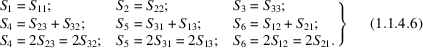The Voigt components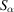form a Voigt matrix: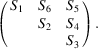The terms of the leading diagonal represent the elongations (see Section 1.3.1). It is important to note that the non-diagonal terms, which represent the shears, are here equal to twice the corresponding components of the strain tensor. The componentsof the Voigt strain matrix are therefore not the components of a tensor. (ii) Stress tensor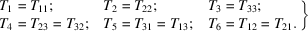The Voigt components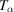form a Voigt matrix: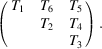The terms of the leading diagonal correspond to principal normal constraints and the non-diagonal terms to shears (see Section 1.3.2).